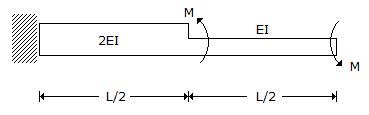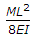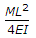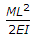# Civil Engineering - GATE Exam Questions

### Exercise :: GATE Exam Questions - Section 7

16.

For a 'best' symmetrical trapezoidal section of an open channel with a given area of section and side slopes, one of the following statements holds true :

 A. Half the top width is equal to one of the side slopes B. Half the top width plus the bottom width is equal to both the side slopes put together C. Water depth is equal to half bottom width D. Hydraulic mean depth is equal to half the top width

Explanation:

No answer description available for this question. Let us discuss.

17.

Temporary hardness in water is caused by the presence of

 A. Bicarbonates of Ca and Mg B. Sulphates of Ca and Mg C. Chlorides of Ca and Mg D. Nitrates of Ca and Mg.

Explanation:

No answer description available for this question. Let us discuss.

18.

Bituminous materials are commonly use in highway construction because of their good

 A. tensile and compression properties. B. binding and water proofing properties. C. shear strength and tensile properties. D. bond and tensile properties.

Explanation:

No answer description available for this question. Let us discuss.

19.

The stepped cantilever is subjected to moments, M as shown in the figure below. The vertical deflection at the free end (neglecting the self weight) isA.B.C.D. Zero

Explanation:

No answer description available for this question. Let us discuss.

20.

A road is provided with a horizontal circular curve having deflection angle of 55° and centre line radius of 250 m. A transition curve is to be provided at each end of the circular curve of such a length that the rate of gain of radial acceleration is 0.3 m/s3 at a speed of 50 km per hour. Length of the transition curve required at each of the ends is

 A. 2.57 m B. 33.33 m C. 35.73 m D. 1666.67 m